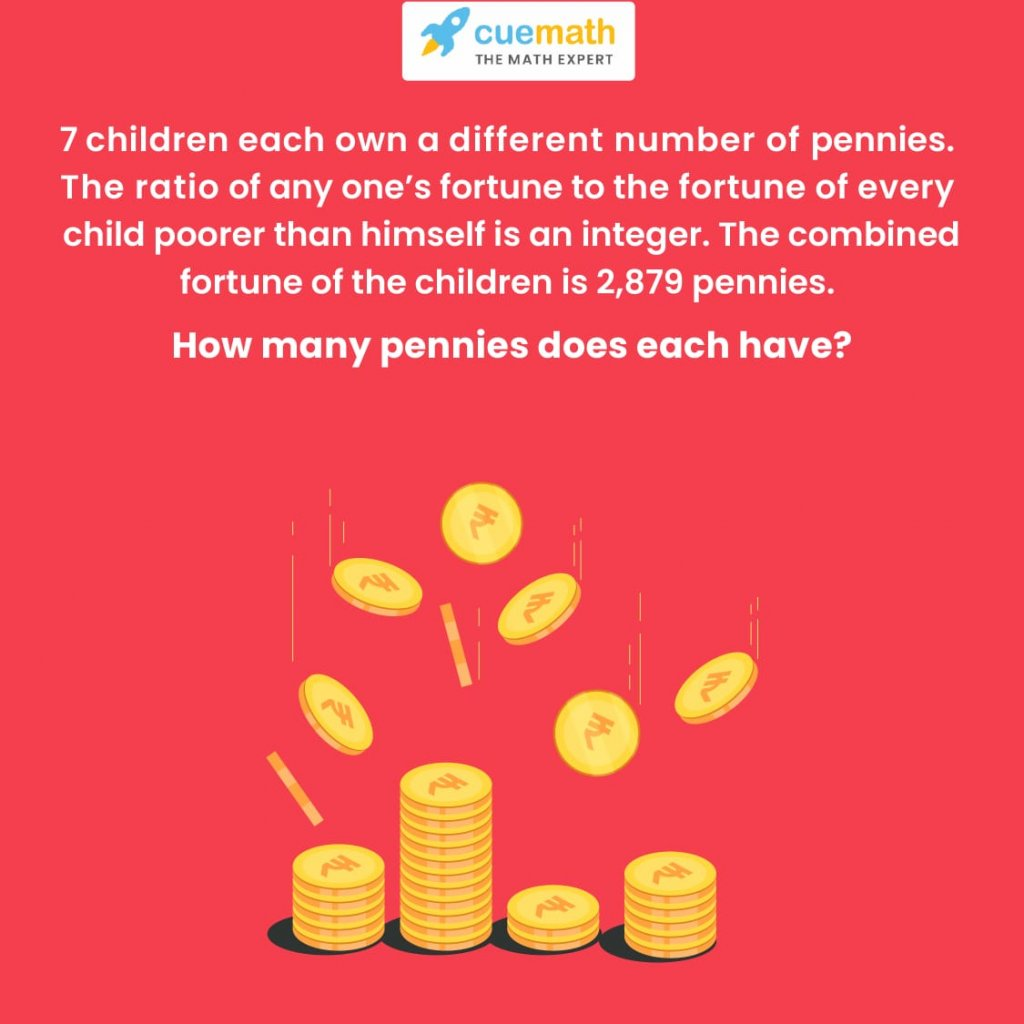Math Puzzles

# Can you solve an interesting prime numbers puzzle?

0

 1 Introduction 2 What is the Solution and Logic for prime numbers puzzle 3 Conclusion 4 FAQs 5 External References

## Introduction

Are you ready to solve an intriguing puzzle on prime numbers?

Prime number puzzles help children not only gain a deeper insight into prime numbers concepts but also strengthen their critical thinking skills for the subject.

Keeping this in mind, we have designed a fun and engaging prime numbers puzzle that will help your child understand the logic for prime number and solving factor puzzles in a fun and engaging way.

This one is called “How many Pennies?” Go ahead! Give this a try!## Can you solve an interesting prime numbers puzzle?

To prove whether a number is a prime number, first try dividing it by 2, and see if you get a whole number. If you do, it can't be a prime number. If you don't get a whole number, next try dividing it by prime numbers: 3, 5, 7, 11 (9 is divisible by 3) and so on, always dividing by a prime number.

Can you solve an interesting prime numbers puzzle? is mentioned below in the Downloadable PDF.

## What is the Solution and Logic for prime numbers puzzle

Here is the solution the the prime number puzzle:

First, let’s assume that the poorest one has x number of pennies.

Now, assume that the next child has the number of pennies equal to xy (where y is an integer)

Next, the ratio of the number of pennies that the third child possesses to the number of pennies possessed by the first two must be integer.

A simple way of achieving this is to assume that the third child has xyz number of pennies (again, z is an integer).This way, the ratios will be:

xyz:xy = z:1           (integer ratio because z is assumed to be an integer)

xyz:x = yz:1           (multiplication of two integers will yield another integer).

Applying the same logic throughout, we can assume the fortunes of other children to be:

4th Child: xyzm           (m is an integer)

5th Child: xyzmn         (n is an integer)

6th Child: xyzmno       (o is an integer)

7th Child: xyzmnop     (p is an integer)

The sum of all fortunes is 2,879.

Mathematically,

x + xy + xyz + xyzm + xyzmn + xyzmno + xyzmnop = 2879

Taking out x as common:

x (1 + y + yz + yzm + yzmn + yzmno + yzmnop) = 2879

Note that 2879 is a prime number – which means that it can be divided only by itself and 1.

In other words, 2879 has only two factors – 1 and 2879.

Therefore, x = 1 and 1 + y + yz + yzm + yzmn + yzmno + yzmnop = 2879

Thus,

y + yz + yzm + yzmn + yzmno + yzmnop = 2879-1

y + yz + yzm + yzmn + yzmno + yzmnop = 2878

Taking y out as common:

(1 + z + zm + zmn + zmno + zmnop) = 2878 = (2)(1439)

Once again, 1439 is a prime number. Therefore, y = 2

Thus,

1 + z + zm + zmn + zmno + zmnop = 1439

z + zm + zmn + zmno + zmnop = 1438

z (1 + m + mn + mno + mnop) = 1438 = (2)(719)

719 is a prime number. Therefore, z = 2

Solving like this, we get:

m = 2, n = 2, o = 2

Finally,

o (1 + p) = 178

(2)(1 + p) = 178

1 + p = 89

p = 88

Thus, the different fortunes will be:

1st Child: 1 penny

2nd Child: 1×2 = 2 pennies

3rd Child: 1x2x2 = 4 pennies

4th Child: 1x2x2x2 = 8 pennies

5th Child: 1x2x2x2x2 = 16 pennies

6th Child: 1x2x2x2x2x2 = 32 pennies

7th Child: 1x2x2x2x2x2x88 = 2816 pennies

## Conclusion

Were you able to solve the tricky maths puzzle?

Either way, hopefully, you enjoyed putting your mind to use for finding the logic for prime number puzzles.

Puzzles are a great way to learn mathematical concepts due to the fun-based learning they provide. It is much more fun to use real life cases or hypothetical situations based on mysterious elements to learn concepts like prime numbers or factoring.

So go ahead and try such prime numbers puzzle and get to solving factor puzzles more. You will definitely have a fun experience.

And better yet, won’t it be cool to ask such puzzles to your friends at school?

Cuemath, a student-friendly mathematics platform, conducts regular Online Live Classes for academics and skill-development and their Mental Math App, on both iOS and Android, is a one-stop solution for kids to develop multiple skills. Know more about the Cuemath fee here, Cuemath Fee

## Which is the smallest odd prime number?

3 is the smallest odd prime number

## External References

To read more articles related to puzzles visit:

Related Articles
Award-winning math curriculum, FREE for a year